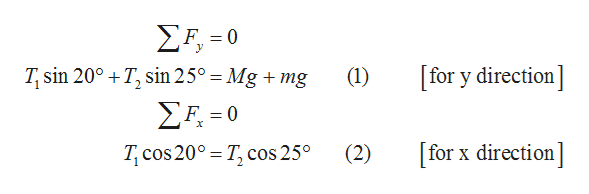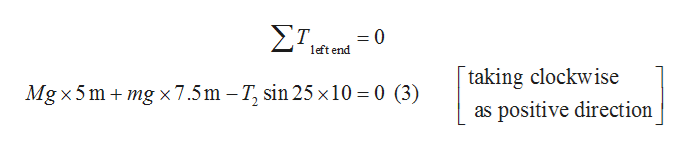# a bridge across a small stream is made by supporting a 10m long log with a mass of 75kg with ropes tied at the ends of the log to the tree on the banks. the rope on the right makes a 25 degree angle with the ground. the rope on the left makes a 20 degree angle with the ground. a 25kg child us standing 7.5 m from the left bank. write the net force equation for the y direction. write the net force equation for the x direction. write the torque equation (use the left end of the log as the axis of rotation).

Question
1 views

a bridge across a small stream is made by supporting a 10m long log with a mass of 75kg with ropes tied at the ends of the log to the tree on the banks. the rope on the right makes a 25 degree angle with the ground. the rope on the left makes a 20 degree angle with the ground. a 25kg child us standing 7.5 m from the left bank. write the net force equation for the y direction. write the net force equation for the x direction. write the torque equation (use the left end of the log as the axis of rotation).

check_circle

Step 1

Let the mass of the log is M and the mass of the child is m. For y direction the torque equation is given by equation (1). The sum of forces acting along x and y direction will be zero.

Step 2

The equilibrium moment about left end of the log is zero.help_outlineImage TranscriptioncloseΣΕ,-0 [for y direction T, sin 20° T, sin 25° = Mg + mg (1) ΣΗ-0 for x direction T cos 20° T, cos 25° (2) fullscreen
Step 3help_outlineImage TranscriptioncloseΣΤ. =0 left end taking clockwise Mg x 5 mmg x 7.5m - T, sin 25 x10 0 (3) as positive direction fullscreen

### Want to see the full answer?

See Solution

#### Want to see this answer and more?

Solutions are written by subject experts who are available 24/7. Questions are typically answered within 1 hour.*

See Solution
*Response times may vary by subject and question.
Tagged in

### Newtons Laws of Motion